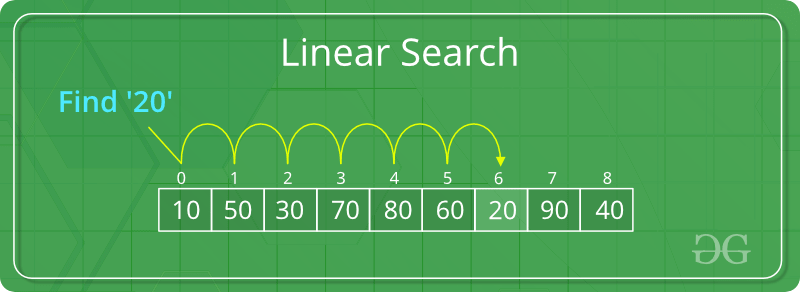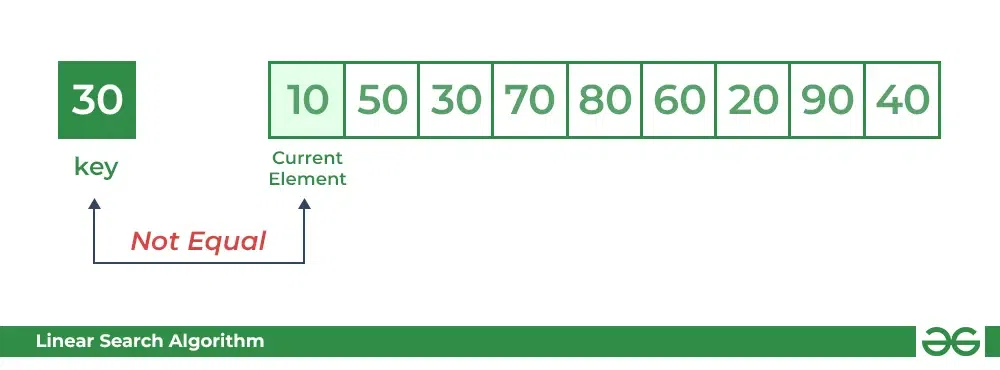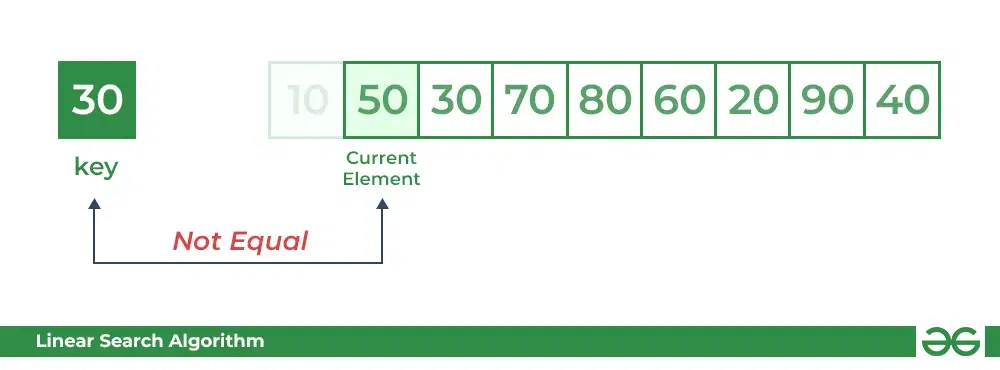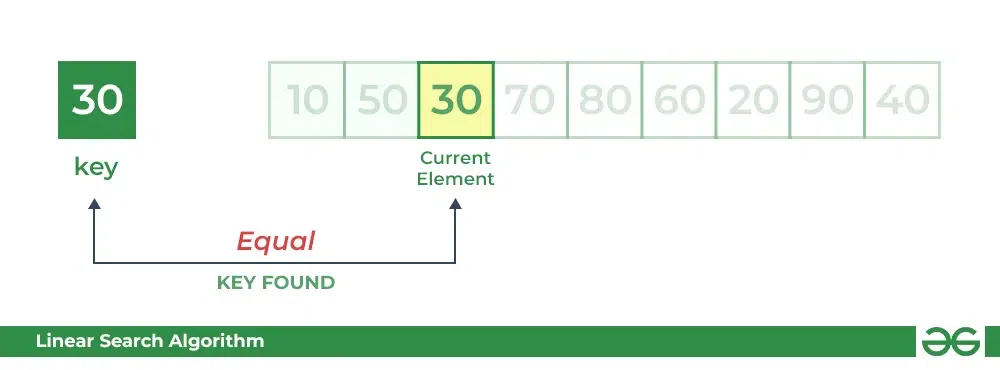GeeksforGeeks App
Open AppBrowser
Continue

# Linear Search Algorithm – Data Structure and Algorithms Tutorials

Linear Search is defined as a sequential search algorithm that starts at one end and goes through each element of a list until the desired element is found, otherwise the search continues till the end of the data set.Linear Search Algorithm

## How Does Linear Search Algorithm Work?

In Linear Search Algorithm,

• Every element is considered as a potential match for the key and checked for the same.
• If any element is found equal to the key, the search is successful and the index of that element is returned.
• If no element is found equal to the key, the search yields “No match found”.

For example: Consider the array arr[] = {10, 50, 30, 70, 80, 20, 90, 40} and key = 30

Step 1: Start from the first element (index 0) and compare key with each element (arr[i]).

• Comparing key with first element arr. SInce not equal, the iterator moves to the next element as a potential match.Compare key with arr

• Comparing key with next element arr. SInce not equal, the iterator moves to the next element as a potential match.Compare key with arr

Step 2: Now when comparing arr with key, the value matches. So the Linear Search Algorithm will yield a successful message and return the index of the element when key is found (here 2).Compare key with arr

## Implementation of Linear Search Algorithm:

Below is the implementation of the linear search algorithm:

## C

 `// C code to linearly search x in arr[].`` ` `#include `` ` `int` `search(``int` `arr[], ``int` `N, ``int` `x)``{``    ``for` `(``int` `i = 0; i < N; i++)``        ``if` `(arr[i] == x)``            ``return` `i;``    ``return` `-1;``}`` ` `// Driver code``int` `main(``void``)``{``    ``int` `arr[] = { 2, 3, 4, 10, 40 };``    ``int` `x = 10;``    ``int` `N = ``sizeof``(arr) / ``sizeof``(arr);`` ` `    ``// Function call``    ``int` `result = search(arr, N, x);``    ``(result == -1)``        ``? ``printf``(``"Element is not present in array"``)``        ``: ``printf``(``"Element is present at index %d"``, result);``    ``return` `0;``}`

## C++

 `// C++ code to linearly search x in arr[].`` ` `#include ``using` `namespace` `std;`` ` `int` `search(``int` `arr[], ``int` `N, ``int` `x)``{``    ``for` `(``int` `i = 0; i < N; i++)``        ``if` `(arr[i] == x)``            ``return` `i;``    ``return` `-1;``}`` ` `// Driver code``int` `main(``void``)``{``    ``int` `arr[] = { 2, 3, 4, 10, 40 };``    ``int` `x = 10;``    ``int` `N = ``sizeof``(arr) / ``sizeof``(arr);`` ` `    ``// Function call``    ``int` `result = search(arr, N, x);``    ``(result == -1)``        ``? cout << ``"Element is not present in array"``        ``: cout << ``"Element is present at index "` `<< result;``    ``return` `0;``}`

## Java

 `// Java code for linearly searching x in arr[]. `` ` `import` `java.io.*;`` ` `class` `GFG {``    ``public` `static` `int` `search(``int` `arr[], ``int` `N, ``int` `x)``    ``{``        ``for` `(``int` `i = ``0``; i < N; i++) {``            ``if` `(arr[i] == x)``                ``return` `i;``        ``}``        ``return` `-``1``;``    ``}`` ` `    ``// Driver code``    ``public` `static` `void` `main(String args[])``    ``{``        ``int` `arr[] = { ``2``, ``3``, ``4``, ``10``, ``40` `};``        ``int` `x = ``10``;`` ` `        ``// Function call``        ``int` `result = search(arr, arr.length, x);``        ``if` `(result == -``1``)``            ``System.out.print(``                ``"Element is not present in array"``);``        ``else``            ``System.out.print(``"Element is present at index "``                             ``+ result);``    ``}``}`

## Python3

 `# Python3 code to linearly search x in arr[].`` ` ` ` `def` `search(arr, N, x):`` ` `    ``for` `i ``in` `range``(``0``, N):``        ``if` `(arr[i] ``=``=` `x):``            ``return` `i``    ``return` `-``1`` ` ` ` `# Driver Code``if` `__name__ ``=``=` `"__main__"``:``    ``arr ``=` `[``2``, ``3``, ``4``, ``10``, ``40``]``    ``x ``=` `10``    ``N ``=` `len``(arr)`` ` `    ``# Function call``    ``result ``=` `search(arr, N, x)``    ``if``(result ``=``=` `-``1``):``        ``print``(``"Element is not present in array"``)``    ``else``:``        ``print``(``"Element is present at index"``, result)`

## C#

 `// C# code to linearly search x in arr[].`` ` `using` `System;`` ` `class` `GFG {``    ``public` `static` `int` `search(``int``[] arr, ``int` `N, ``int` `x)``    ``{``        ``for` `(``int` `i = 0; i < N; i++) {``            ``if` `(arr[i] == x)``                ``return` `i;``        ``}``        ``return` `-1;``    ``}`` ` `    ``// Driver's code``    ``public` `static` `void` `Main()``    ``{``        ``int``[] arr = { 2, 3, 4, 10, 40 };``        ``int` `x = 10;`` ` `        ``// Function call``        ``int` `result = search(arr, arr.Length, x);``        ``if` `(result == -1)``            ``Console.WriteLine(``                ``"Element is not present in array"``);``        ``else``            ``Console.WriteLine(``"Element is present at index "``                              ``+ result);``    ``}``}`` ` `// This code is contributed by DrRoot_`

## PHP

 ``

## Javascript

 `// Javascript code to linearly search x in arr[].`` ` `function` `search(arr, n, x)``{``    ``for` `(let i = 0; i < n; i++)``        ``if` `(arr[i] == x)``            ``return` `i;``    ``return` `-1;``}`` ` `// Driver code`` ` `    ``let arr = [ 2, 3, 4, 10, 40 ];``    ``let x = 10;``    ``let n = arr.length;`` ` `    ``// Function call``    ``let result = search(arr, n, x);``    ``(result == -1)``        ``? console.log(``"Element is not present in array"``)``        ``: console.log(``"Element is present at index "` `+ result);`` ` `// This code is contributed by Manoj`

Output

`Element is present at index 3`

## Complexity Analysis of Linear Search:

Time Complexity:

• Best Case: In the best case, the key might be present at the first index. So the best case complexity is O(1)
• Worst Case: In the worst case, the key might be present at the last index i.e., opposite to the end from which the search has started in the list. So the worst-case complexity is O(N) where N is the size of the list.
• Average Case: O(N)

Auxiliary Space: O(1) as except for the variable to iterate through the list, no other variable is used.

• Linear search can be used irrespective of whether the array is sorted or not. It can be used on arrays of any data type.
• Does not require any additional memory.
• It is a well-suited algorithm for small datasets.

## Drawbacks of Linear Search:

• Linear search has a time complexity of O(N), which in turn makes it slow for large datasets.
• Not suitable for large arrays.

## When to use Linear Search?

• When we are dealing with a small dataset.
• When you are searching for a dataset stored in contiguous memory.

My Personal Notes arrow_drop_up